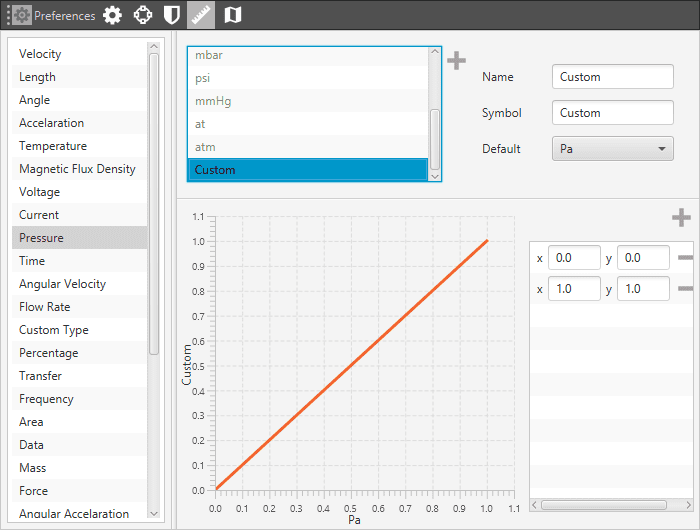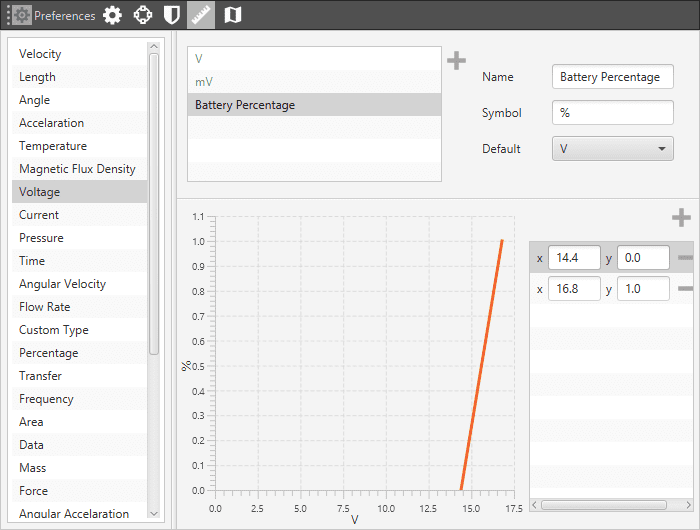# System Units¶

This panel shows all the system units available for the system variables. They are arranged according to the type of variable (velocity, acceleration, temperature, etc). It can be found in PreferencesUnits.System Units

Veronte Pipe works by default with units of the International System, but these ones can be changed by selecting others from the ones shown when pressing Default option.

## Creating Custom Units¶

1. Click on “+” button, and a new variable will appear in the menu.

2. Introduce the conversion from the default unit to the new one. The conversion formula is created by introducing as many points as desired in the graph shown in the next figure.Custom Unit Definition

An example is added to improve the understanding:

Considering the voltage of a LiPo battery, it could be useful to have a unit that indicates the remaining percentage of battery. For example, if the battery used is a 4S, the fully charged voltage is 16.8 V, while 14.4 will be the one when the battery does not provide the amount of energy needed to fly. So 16.8 V will correspond to 100% and 14.4 to 0%. Taking that into account the conversion graph will be the one shown in the next figure.Voltage To Percentaje Conversion

Warning

In this panel, it can be selected the default system units. It means the first displayed unit will be the selected one, but it will be possible to change it temporarily in all Pipe windows.

## Units¶

The table below shows all the available units in VerontePipe.

Variable Type

Units

Velocity

[m/s] [kt] [km/h] [mph] [ft/s] [mm/s] [ft/m]

Length

[m] [km] [mi] [NM] [yd] [ft] [in] [cm] [mm]

Angle

rad[-π;π]°[-180;180] rad[0;2π] °[0;360] [° ‘ “] [° ‘ “](N/S) [° ‘ “](E/W)

Acceleration

[m/s²] [ft/s²] [in/s²] [g]

Temperature

[K] [°C] [°F]

Magnetic Flux Density

[T] [mG] [gauss] [nT]

Voltage

[V] [mV]

Current

[A] [mA]

Pressure

[Pa] [kPa] [bar] [mbar] [psi] [mmHg] [at] [atm]

Time

[s] [min] [h] [μs] [ms] [Time]

Angular Velocity

Flow Rate

[m³/s] [gal/s] [gal/h] [l/s] [l/h]

Custom Type

[- -]

Percentage

[x1] [%]

Transfer

[pkts/s]

Frequency

[Hz] [mHz] [kHz]

Area

[m²] [cm²] [mm²] [km²] [mile²] [ft²] [yd²]

Data

[bit] [byte] [KB] [GB] [bytes/s]

Mass

[kg] [g] [tonnes] [lbs] [oz]

Force

[N] [kN] [lbf] [pdl]

Angular Acceleration

Baudrate

[Bd] [kBd] [MBd]

Pressure Variance

[Pa²]

Magfield Variance

[T²]

Velocity Variance

[(m/s)²] [(cm/s)²] [(mm/s)²]

Numeral System

[bin] [octal] [dec] [hex]

Pressure Square Error Rate

[Pa²/s]

Centimeters/Pixels

[cm/pixel]

Jerk

[m/s³]

Power

[W] [kW] [Kgm/s] [erg/s] [CV]